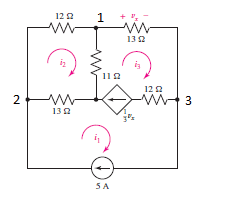# Solving a circuit using both the mesh and node analysis

• kstorm19
In summary, the student attempted to solve for i1, i2, and i3 using the mesh method in the following circuit: 1. The problem asks to solve for i1, i2, and i3 using the mesh method in the following circuit.2. First, the student solved for all three currents using the mesh analysis.3. For mesh 2, this is the equation I came up with:12i2 + 11(i2-i3) + 13 (i2-i1) = 04. And so, using the Node 3:i3 = 5+ 1/3 VxBut Vx = 13i3Sof

## Homework Statement

The problem asks to solve for i1, i2, and i3 using the mesh method in the following circuit:I will also include the link, because I noticed I am having trouble attaching the image to the post: https://imgur.com/a/bBvuLXMI would also like to confirm the answer by solving using the node analysis.2. Homework Equations

V=IR

## The Attempt at a Solution

First of all, I solved for all three currents using the mesh analysis.
i1 = 5 A
For mesh 2, this is the equation I came up with:
12i2 + 11(i2-i3) + 13 (i2-i1) = 0

And so, using the Node 3:
i3 = 5+ 1/3 Vx
But Vx = 13i3
So i3 = 5 + 13/3 i3
i3= -1.5 A

Going back to equation for mesh 2 and solving for i2:
i2= -1.35 A

So I have:
i1 = 5 A
i2= -1.35 A
i3= -1.5 A

I wanted to confirm these results using the node analysis.

For node 1

(V1-V2)/12 + V1/11 + (V1-V3)/13 = 0

For node 2

(V2-V1)/12 + V2/13 = 5

For node 3

(V3-V1)/13 + 1/3 Vx + 5 = 0

So in this last equation I got a little confused, since when I was setting it up, V3 has the highest potential. If Vx = V1-V3 by looking at the arrow, should I write it that way in the equation, or should I put instead V3-V1?

I figured that maybe it should be written using V3-V1, so this is how I wrote the equation for node 3:

(V3-V1)/13 + 1/3 (V3-V1) + 5 = 0

Solving these 3 equations, I got:

V1 = 6.036 V
V2 = -0.061 V
V3 = -18.23 V

Now, replacing for Vx, which should be -19.5 V since Vx = 13i3 and i3=-1.5 A, I don't get that answer.

I also tried (V3-V1)/13 + 1/3 (V1-V3) + 5 = 0 for the third node equation in case I was wrong and I got:

V1 = -6.64 V
V2 = -3.21 V
V3 = 12.58 V

Now, subtracting V1-V3 gets me -19.22 V (almost -19.5 V from the mesh analysis).
But looking to confirm that i2=-1.35 A, (V2-V1)/12 doesn´t get me anywhere near that value. Where did I go wrong?

Thank you so much guys for your time and help.

#### Attachments

Last edited by a moderator:
So in this last equation I got a little confused, since when I was setting it up, V3 has the highest potential. If Vx = V1-V3 by looking at the arrow, should I write it that way in the equation, or should I put instead V3-V1?
From the circuit diagram, ##V_x = V1 - V3##

From the circuit diagram, ##V_x = V1 - V3##

Thank you for clearing my doubt in this part of the problem! I would also like to thank the mod that included my image in the post.

So now I know the second set of equations is the correct one. It is consistent with V1-V3 being -19.5V as Vx=13i3 is -19.5 V as well. What I'm not so sure about is the second current (i2), it definitely doesn´t get me the value that I got using the mesh analysis. What am I missing here? Thank you so much guys for your help.

I think that the problem must lie somewhere in your work in solving the simultaneous equations. You'll have to show the step-by-step details for us to critique it.